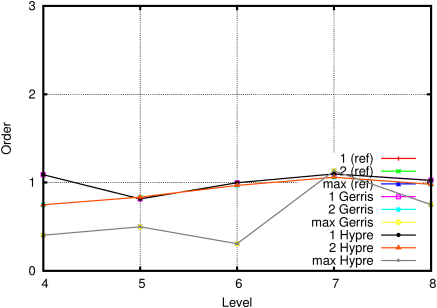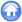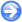### 2.1PASS: Convergence of the Poisson solver

Author
Stéphane Popinet
Command
sh poisson.sh poisson.gfs
Version
100325
Required files
poisson.sh res-7.ref error.ref order.ref
Running time
52 seconds

This is one of the test cases presented in Popinet . We solve the Poisson equation in a square domain with Dirichlet boundary conditions on all sides and the right-hand-side:

 ∇·U⋆⋆(x,y) = −π2(k2+l2)sin(π kx)sin(π ly)

with k = l = 3. The exact solution of the Poisson equation with this source term is

 φ(x,y)=sin(π kx)sin(π ly).

Figure 1 illustrates the evolution of the maximum residual as a function of CPU time. Figure 2 illustrates the average residual reduction factor (per V-cycle). The evolution of the norms of the error of the final solution as a function of resolution is illustrated on Figure 3. The corresponding order of convergence is given on Figure 4.

The curves labeled "Hypre" were obtained using the hypre module solver rather than Gerris’ built-in multilevel solver.

 Figure 1: Evolution of the residual.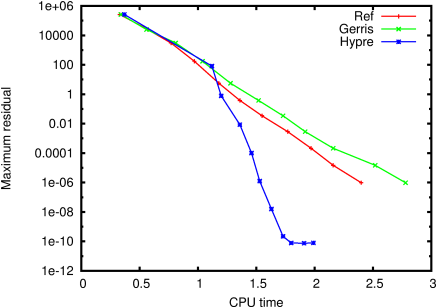Figure 2: Average reduction factor.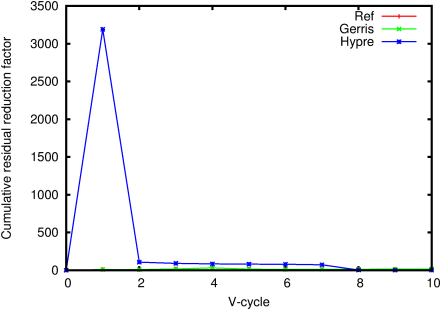Figure 3: Evolution of the error as a function of resolution.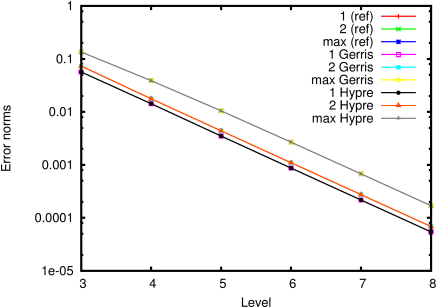Figure 4: Corresponding convergence order.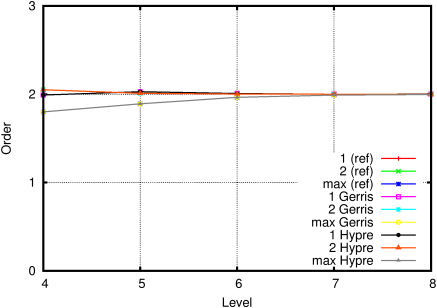#### 2.1.1PASS: Convergence with a refined circle

Author
Stéphane Popinet
Command
sh ../poisson.sh circle.gfs
Version
100325
Required files
res-7.ref error.ref order.ref
Running time
3 minutes 33 seconds

Same as the previous test but in order to test the accuracy of the gradient operator at coarse/fine boundaries, two levels of refinement are added in a circle centered on the origin and of radius 0.25.

The solver still shows second-order accuracy in all norms (Figure 8).

 Figure 5: Evolution of the residual.Figure 6: Average reduction factor.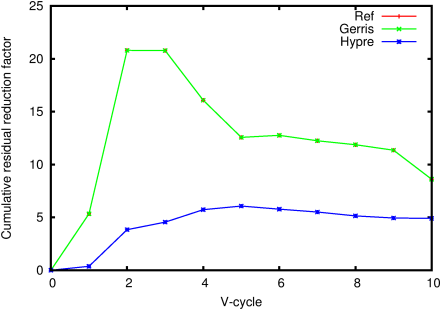Figure 7: Evolution of the error as a function of resolution.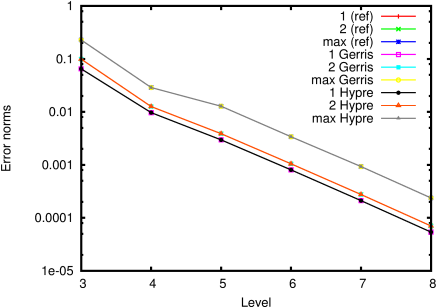Figure 8: Corresponding convergence order.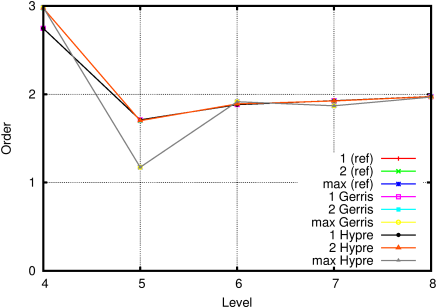#### 2.1.2PASS: Dirichlet boundary condition

Author
Stéphane Popinet
Command
sh ../poisson.sh dirichlet.gfs
Version
100707
Required files
res-7.ref error.ref order.ref
Running time
36 seconds

Similar to the previous test but with embedded solid boundaries and a non-trivial Dirichlet boundary condition. This test case was proposed by . The boundary of the domain is defined by

 r < 0.30 + 0.15cos(6θ)

The Poisson problem to solve in this domain is

 ∇2Φ=7r2cos(3θ)

which has the exact solution

 Φ=r4cos(3θ)

which is used as boundary condition.

 Figure 9: Evolution of the residual.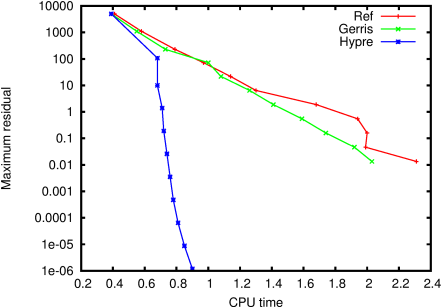Figure 10: Average reduction factor.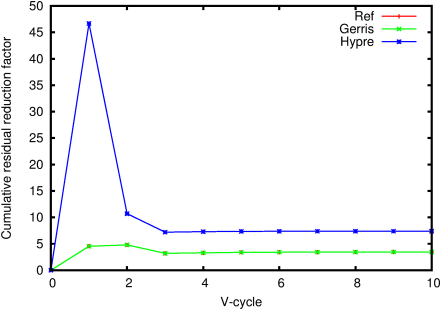Figure 11: Evolution of the error as a function of resolution.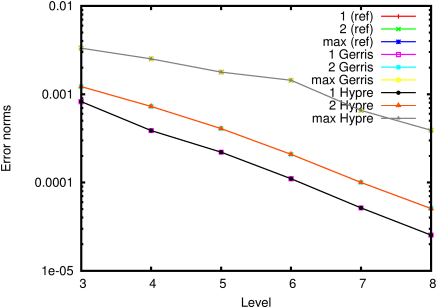Figure 12: Corresponding convergence order.